﻿ 行星会合指数与行星系质心的日心经度模型构建及数值模拟
 地球物理学进展2017, Vol. 32Issue (2): 506-515PDF

1. 南京师范大学数学科学学院地理科学学院, 南京 210023
2. 安庆师范大学资源环境学院, 安庆 246133
3. 江苏省地理信息资源开发与利用协同创新中心, 南京 210023
4. 同济大学经济与管理学院, 上海 201804
5. 齐齐哈尔大学理学院地理系, 齐齐哈尔 161006

Modeling of the planet juncture index and heliocentric longitude of the centroid of planetary systems and numerical simulation
SUN Wei1,2,3, WANG Jian1,3, CHEN Jin-ru1,3 , SUN Li4 , ZHOU Bao-hua2 , LIU Fu-gang5
1. College of MathematicsCollege of Geography, Nanjing Normal University, Nanjing 210023, China
2. School of Resources and Environment, Anqing Normal University, Anqing 246133, China
3. Jiangsu Center for Collaborative Innovation in Geographical Information Resource Development and Application, Nanjing 210023, China
4. School of Economics and Management, Tongji University, Shanghai 201804, China
5. College of Science, Qiqihar University, Qiqihar 161006, China
Abstract: In this article, the kinematic equation of the heliocentric longitude was created based on the orbital elements of the planets in the solar system, which can be used to investigate the periodic movement of the sun around the mass center of the solar system. The sidereal period of the Sun around the centroid of solar system was obtained as 11.5 years by the way of numerical simulation. Both of the kinematic equation of the heliocentric longitude and the kinematic equation of the planet juncture index were studied by simulation. Then, both of the trajectory of the Sun around the solar system barycenter and the cycle of 11.5 years were also obtained by numerical simulation. On analysis of the date of the positions between the Sun and the solar system barycenter for 1000 years, we found that about one-third of the time of the centroid of solar system was in the body of the Sun during the track.
Key words: heliocentric longitude     kinematics equation     centroid of planetary system     planet juncture index     numerical simulation
0 引言

1 行星系日心经度运动学方程的建立 1.1 太阳系行星系统质心的确定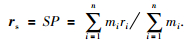(1)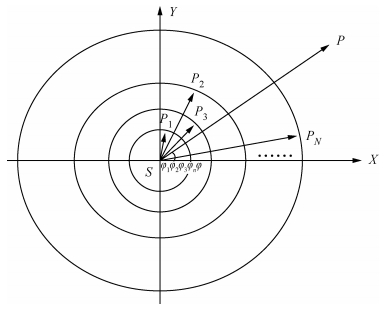图 1 行星会合示意图 Figure 1 The sketch map of planetary rendezvous表 1 太阳系行星轨道有关天文参数 Table 1 The astronomical data about the planetary orbits
1.2 行星系质心绕日会合指数运动学方程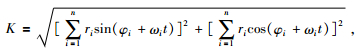(2)表 2 2000年1月1日12时八大行星的日心经度 (φi)(J2000历年，世界时) Table 2 The heliocentric longitude of the eight planet at 12 o'clock on January 1st, 2000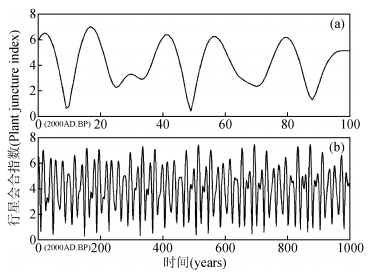图 2 行星系统质心P绕日S的会合指数图 (步长为1年) Figure 2 The juncture index of planetary system mass center (P) orbiting the fixed point of the sun (S)(the time unit was 1-year)

1.3 行星系日心经度运动学方程的构建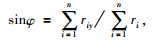(3)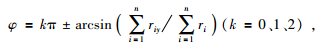(4)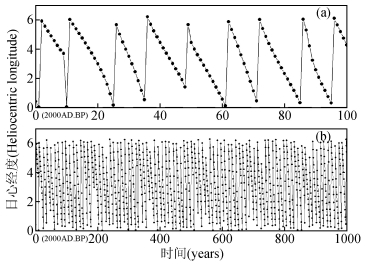图 3 行星系统质心日心经度图 (步长为1年) Figure 3 The heliocentric longitude of planetary system mass center (P) orbiting the fixed point of the sun (S) (the time unit was 1-year)

2 行星系质心与太阳质心运动轨迹的数值模拟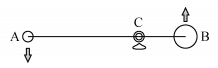图 4 行星系质心和太阳质心绕太阳系质心的运转图 Figure 4 Both of the centroid of planetary system and the sun moving around the mass center of the solar system
2.1 行星系质心绕太阳质心运动轨迹的数值模拟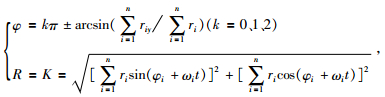(5)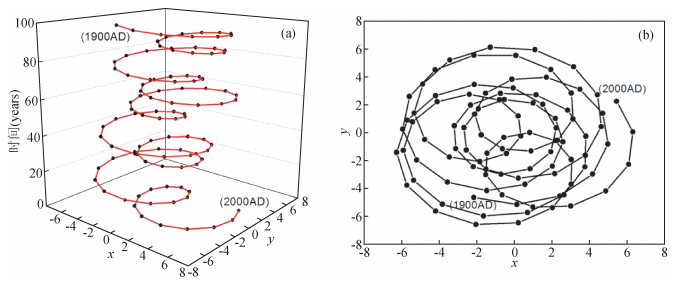图 5 行星系质心绕日运动轨迹图 Figure 5 Trajectory of the centroid of planetary system relative to the sun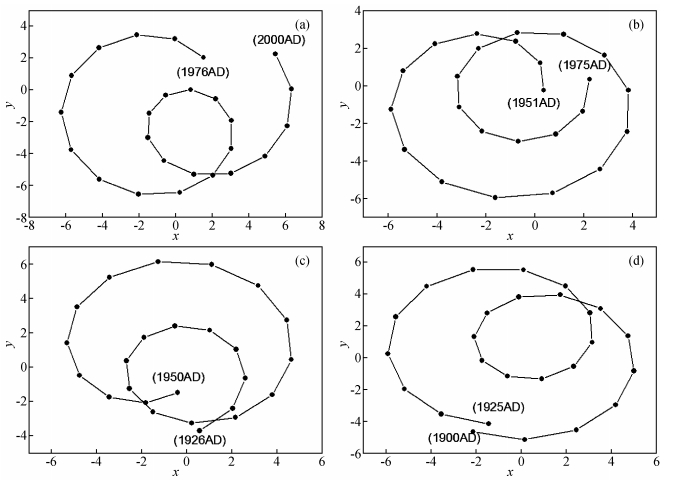图 6 行星系质心绕日运动轨迹图 Figure 6 Trajectory of the centroid of planetary system relative to the sun
2.2 太阳质心与太阳系质心相互运动轨迹的数值模拟 2.2.1 太阳质心绕太阳系质心运动轨迹的数值模拟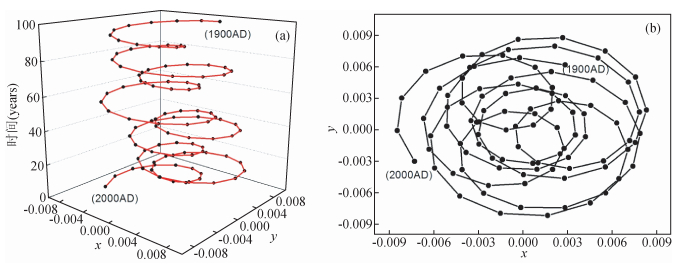图 7 太阳质心绕太阳系质心运动轨迹图 Figure 7 Trajectory of the Sun center relative to the mass center of the solar system
2.2.2 太阳系质心相对于太阳质心运动轨迹的数值模拟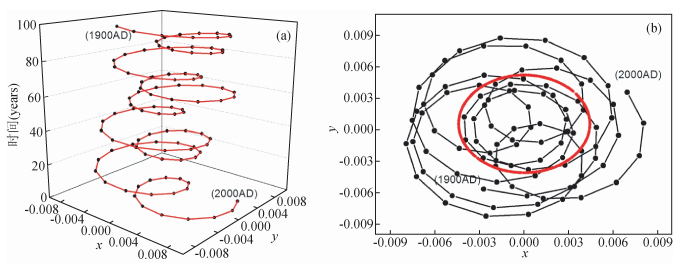图 8 太阳系质心相对于太阳质心运动轨迹图 Figure 8 Trajectory of the mass center of the solar system relative to the Sun表 3 太阳系质心与太阳本体位置关系的统计表 Table 3 Statistics relating to the position relationship of the solar system barycenter and the sun ontology.

3 不同模型数值模拟结果对比与分析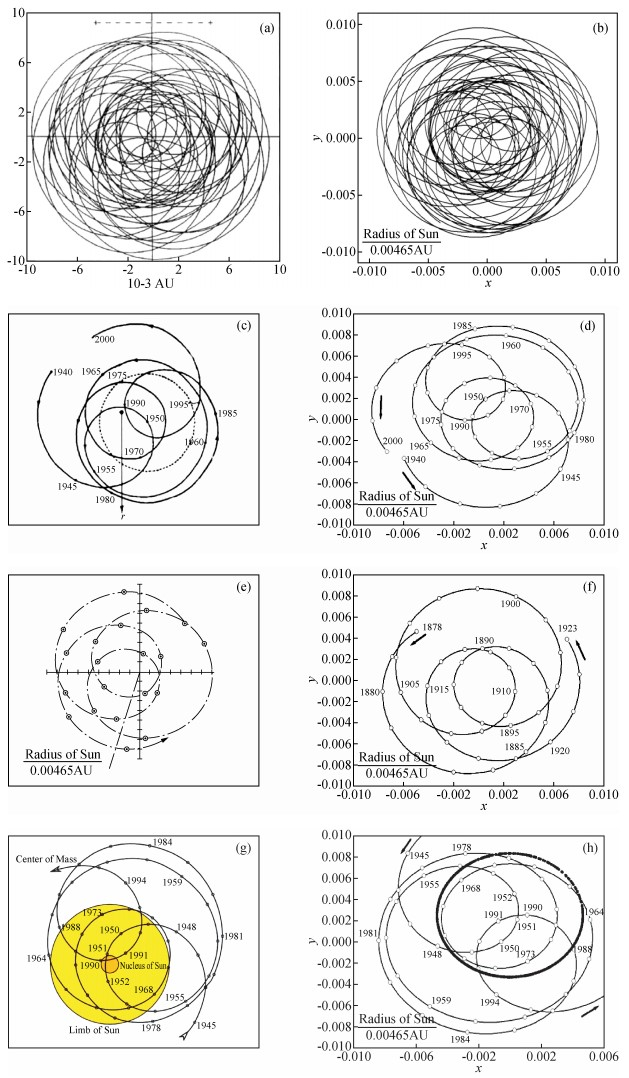图 9 不同模型的数值模拟结果对比图 Figure 9 Numerical simulation of different models.
4 结论 4.1

4.2

4.3

4.4

4.5

 [] Charvátová I. 2000. Can origin of the 2400-year cycle of solar activity be caused by solar inertial motion?[J]. Annales Geophysicae, 18(4): 399–405. DOI:10.1007/s00585-000-0399-x [] Chen X Z. 1996. Solar activity modulation by planetary perturbations[J]. Acta Astrophysica Sinica, 16(2): 207–212. [] Dai W S. 1979. The Development of Solar System (Rudin)[M]. Shanghai: Shanghai Scientific & Technical Publishers: 22-23. [] Damon P E, Cheng S L, Linick T W. 1989. Fine and hyperfine structure in the spectrum of secular variations of atmospheric 14C[J]. Radiocarbon, 31(3): 704–718. DOI:10.1017/S0033822200012303 [] Damon P E, Linick T W. 1986. Geomagnetic-heliomagnetic modulation of atmospheric radiocarbon production[J]. Radiocarbon, 28(2A): 266–278. DOI:10.1017/S0033822200007360 [] Friis-Christensen E, Lassen K. 1991. Length of the solar cycle:An indicator of solar activity closely associated with climate[J]. Science, 254(5032): 698–700. DOI:10.1126/science.254.5032.698 [] Haigh J D. 1996. The impact of solar variability on climate[J]. Science, 272(5264): 981–984. DOI:10.1126/science.272.5264.981 [] Hood L L, Jirikowic J L. 1991. A probable approx. 2400 year solar quasi-cycle in atmospheric delta 14C[J]. Holocene, 12: 98–105. [] Jose P D. 1965. Sun's motion and sunspots[J]. The Astronomical Journal, 70(3): 193–200. [] Li K J, Feng W, Liang H F. 2010. The abnormal 24th solar cycle-The first complete solar cycle of the new millennium[J]. Scientia Sinica Physics, Mechanics & Astronomy, 40(10): 1293–1301. [] Liu F G, Wang J. 2013. Changes of the planet juncture index and solar revolution cycle around the mass center of the solar system[J]. Chinese Journal of Geophysics, 56(5): 1457–1466. DOI:10.6038/cjg20130504 [] Liu F G, Wang J, Bai S B, et al. 2013a. The planetary motion and the change of 22-year cycle of the Sun spin velocity[J]. Progress in Geophysics, 28(4): 1678–1683. DOI:10.6038/pg20130406 [] Liu F G, Wang J, Shang Z Y, et al. 2013b. Study on long-term cyclical rhythm of solar activity[J]. Progress in Geophysics, 28(2): 570–578. DOI:10.6038/pg20130205 [] Liu L B, Wan W X, Chen Y D, et al. 2011. Solar activity effects of the ionosphere:A brief review[J]. Chinese Science Bulletin, 56(12): 1202–1211. DOI:10.1007/s11434-010-4226-9 [] Qu W Z, Deng S G, Huang F, et al. 2004. Influence of magnetic index abnormal change in the solar magnetic field on climate at the middle latitudes of North Hemisphere[J]. Chinese Journal of Geophysics, 47(3): 398–404. DOI:10.3321/j.issn:0001-5733.2004.03.005 [] Qu W Z, Huang F, Zhao J P, et al. 2007. The significance of solar magnetic field direction variation on anomalous variability of the atmosphere temperature on the earth[J]. Chinese Journal of Geophysics, 50(5): 1304–1310. DOI:10.3321/j.issn:0001-5733.2007.05.005 [] Sonett C P, Finney S A, Berger A. 1990. The spectrum of radiocarbon[J]. Philos. Trans. Roy. Soc. A Math. Phys. Eng. Sci., 330(1615): 413–426. DOI:10.1098/rsta.1990.0022 [] Suess H E. 1980. The radiocarbon record in tree rings of the last 8000 years[J]. Radiocarbon, 22(2): 200–209. DOI:10.1017/S0033822200009462 [] Sun W, Wang J, Chen J R, et al. 2017. Variations of the planet juncture index and heliocentric longitude with spectral analysis for approximately 2000 years[J]. Chinese Science Bulletin, 62(5): 407–419. DOI:10.1360/N972016-00447 [] Tlatov A G. 2007. 22-year variations of the solar rotation and solar activity cycles[J]. Astronomy Letters, 33(11): 771–779. DOI:10.1134/S1063773707110084 [] Ren Z Q. 1990. Global Change[M]. Beijing: Science Press: 23. [] Yang D H, Yang X X. 2013. Study and model on variation of Earth's Rotation speed[J]. Progress in Geophysics, 28(1): 58–70. DOI:10.6038/pg20130107 [] Yang R W, Cao J, Huang W, et al. 2009. Cross wavelet analysis of the relationship between total solar irradiance and sunspot number[J]. Chinese Science Bulletin, 54(7): 871–875. [] Yang Z G. 1991. The possible influence of the orbital motion of the major planets on sunspot activities[J]. Acta Astrophysica Sinica, 11(4): 370–377. [] Yang Z G, Zhao M. 1988. An investigation for the cause of formation of the major period of the relative sunspot numbers with bipolarity[J]. Acta Astronomica Sinica, 29(3): 297–304. [] Zhao M Y, Chen J Q, Liu Y, et al. 2014. Statistical analysis of sunspot groups and flares for solar maximum and minimum[J]. Scientia Sinica Physics, Mechanics & Astronomy, 44(1): 109–120. [] Zhao P, Zhou X J, Liu G. 2011. Decadal-centennial-scale change in Asian-Pacific summer thermal contrast and solar activity[J]. Chinese Science Bulletin, 56(28-29): 3012–3018. DOI:10.1007/s11434-011-4591-z [] Zhao X H, Feng X S. 2014. Periodicities of solar activity and the surface temperature variation of the Earth and their correlations[J]. Chinese Science Bulletin, 59(14): 1284–1292. DOI:10.1360/972013-1089 [] Zhou Y B. 1986. The Course of Theoretical Mechanics[M]. 2nd ed. Beijing: Higher Education Press: 112-114. [] 陈协珍. 1996. 行星摄动力对太阳活动的调制[J]. 天体物理学报, 16(2): 207–212. [] 戴文赛. 1979. 太阳系演化学 (上册)[M]. 上海: 上海科技出版社: 22-23. [] 李可军, 冯雯, 梁红飞. 2010. 异常的第24太阳活动周——新千年的第一个完整的太阳活动周[J]. 中国科学:物理学力学天文学, 40(10): 1293–1301. [] 刘复刚, 王建. 2013. 行星会合指数变化与太阳绕太阳系质心运转的周期[J]. 地球物理学报, 56(5): 1457–1466. DOI:10.6038/cjg20130504 [] 刘复刚, 王建, 白世彪, 等. 2013a. 行星运动与太阳自转角速度22年周期变化[J]. 地球物理学进展, 28(4): 1678–1683. DOI:10.6038/pg20130406 [] 刘复刚, 王建, 商志远, 等. 2013b. 太阳轨道运动长周期性韵律的成因[J]. 地球物理学进展, 28(2): 570–578. DOI:10.6038/pg20130205 [] 刘立波, 万卫星, 陈一定, 等. 2011. 电离层与太阳活动性关系[J]. 科学通报, 56(7): 477–487. [] 曲维政, 邓声贵, 黄菲, 等. 2004. 太阳磁场磁性指数异常变化对南北半球中纬度气候的影响[J]. 地球物理学报, 47(3): 398–404. DOI:10.3321/j.issn:0001-5733.2004.03.005 [] 曲维政, 黄菲, 赵进平, 等. 2007. 太阳磁场方向变化对于地球大气温度异常变化的意义[J]. 地球物理学报, 50(5): 1304–1310. DOI:10.3321/j.issn:0001-5733.2007.05.005 [] 任振球. 1990. 全球变化:地球四大圈异常变化及其天文成因[M]. 北京: 科学出版社: 23. [] 孙威, 王建, 陈金如, 等. 2017. 近两千年以来行星会合指数与行星系日心经度变化及频谱分析[J]. 科学通报, 62(5): 407–419. [] 杨东红, 杨学祥. 2013. 地球自转速度变化规律的研究和计算模型[J]. 地球物理学进展, 28(1): 58–70. DOI:10.6038/pg20130107 [] 杨若文, 曹杰, 黄玮, 等. 2009. 太阳常数与太阳黑子数关系的交叉小波分析[J]. 科学通报, 54(7): 871–875. [] 杨志根. 1991. 大行星运动对太阳黑子活动的可能影响[J]. 天体物理学报, 11(4): 370–377. [] 杨志根, 赵铭. 1988. 太阳极性黑子相对数主周期的成因探讨[J]. 天文学报, 29(3): 297–304. [] 赵明宇, 陈军权, 刘煜, 等. 2014. 太阳活动峰年和谷年期间黑子群与耀斑的统计分析[J]. 中国科学:物理学力学天文学, 44(1): 109–120. [] 赵平, 周秀骥, 刘舸. 2011. 夏季亚洲-太平洋热力差异年代-百年尺度变化与太阳活动[J]. 科学通报, 56(25): 2068–2074. [] 赵新华, 冯学尚. 2014. 太阳活动与地球表面温度变化的周期性和相关性[J]. 科学通报, 59(14): 1284–1292. [] 周衍柏. 1986. 理论力学教程[M]. 2版. 北京: 高等教育出版社: 112-114.